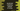# How to use the substring method in JavaScript string## How to use the substring method in JavaScript string:

The substring() method can be used to get a part of string or substring in between two indexes. We can get a substring between two given indexes of we can get a substring from a string to the end of the string.

In this post, we will learn how to use this method with its definitions and examples.

### Definition of substring:

The substring method is defined as like below:

``substring(start, end)``

Here,

• start is the start index. It is the index of the start character from where we are building the substring.
• end is the end index. It is an optional value. It is the index after the end character index for the substring.

This method returns a new substring from start to end - 1 index of the original string.

### Note:

• end is an optional value. If we don’t provide this value, it will extract the substring to the end of the string.
• If start is equal to end, it will return an empty string.
• If start or end is NaN, it will be treated as 0.
• If start is less than 0, it is considered as 0. Similarly, if end is greater than string length i.e. end index, it is considered as string length.
• If start is greater than end, it will be as like the arguments are swapped.

### Example 1: Example of substring():

Let’s take a look at the below example:

``````const givenStr = "HelloWorld";

console.log(givenStr.substring(5, 7));
console.log(givenStr.substring(5));``````

In this example,

• HelloWorld is the given string. The index starts with 0 in a string i.e. the index of the first character is 0, the index of the second character is 1 etc.
• The first statement creates a substring from index 5 to 6. So, it will print Wo.
• The second statement creates a substring from index 5 to the end of the string, because the end index is not provided. So, it will print World.

### Example 2: Example of substring with start or end as NaN:

Let’s check how it works if any of start or end index is NaN:

``````const givenStr = "HelloWorld";

console.log(givenStr.substring(NaN, 7));
console.log(givenStr.substring(5, NaN));``````

It will print:

``````HelloWo
Hello``````

### Example 3: start is equal to end:

For the below example, start is equal to end:

``````const givenStr = "HelloWorld";

console.log(givenStr.substring(7, 7));
console.log(givenStr.substring(0, 0));``````

It will print empty strings for both.

### Example 4: start is greater than end:

For the below example, start is greater than end:

``````const givenStr = "HelloWorld";

console.log(givenStr.substring(8, 5));
console.log(givenStr.substring(5, 2));``````

If the value of start is greater than end, it will create a substring from end to start - 1. The above program will print:

``````Wor
llo``````

### Example 5: start less than 0 and end greater than the length:

If the value of start is less than 0 and if the value of end is greater than the string length, it will start from the first character and ends at the end of the string.

``````const givenStr = "HelloWorld";

console.log(givenStr.substring(-10, 5));
console.log(givenStr.substring(5, 2000));``````

For the first one, it will create a substring from index 0 to 4. For the second one, it will create a substring from index 5 to the end.

It will print:

``````Hello
World``````